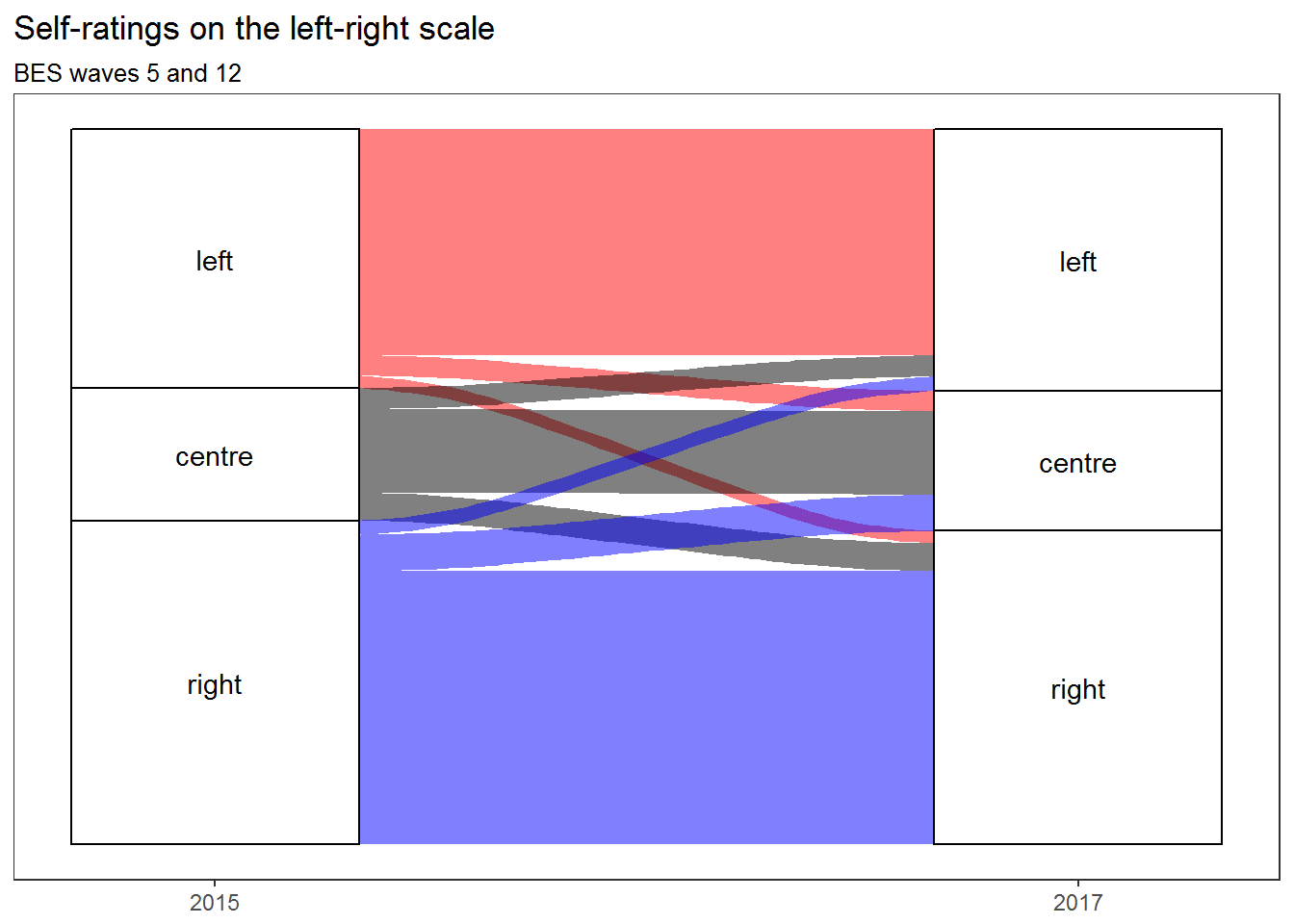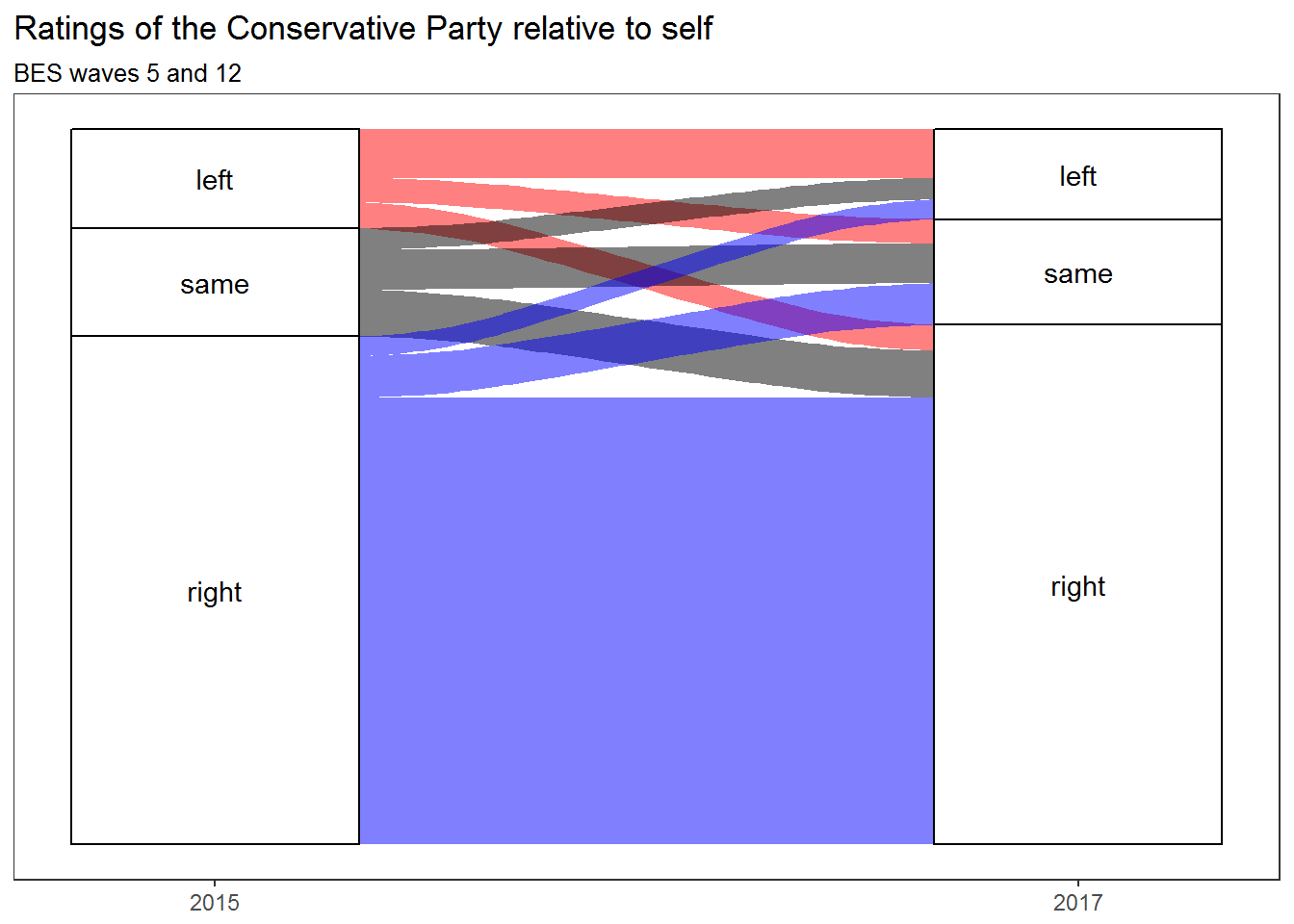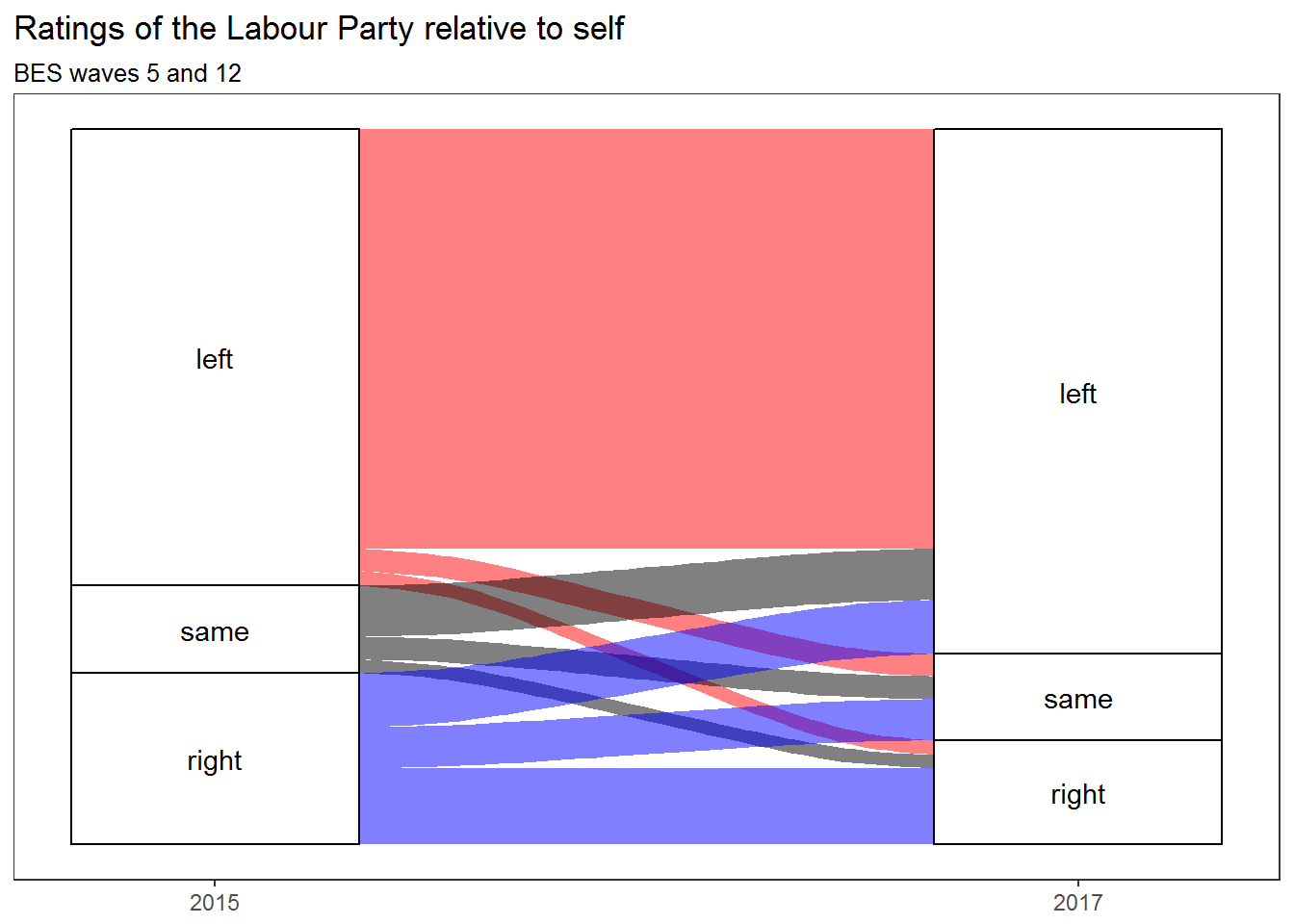This document explains how to create the alluvial diagrams I used in my blog post on how British voters’ perceptions of themselves and the UK’s two main parties have shifted around the left-right scale, as well as presenting the raw and weighted figures and discussing what they mean. There’s an entry-level ggaluvial tutorial buried in here.

# load data
# retain only individuals who participated in both wave 5 and wave 12
d <- filter(d, !is.na(wt_full_W5), !is.na(wt_new_W12))
## Warning: package 'bindrcpp' was built under R version 3.3.3
# retain only the variables we need: left-right rating of self and the two main
# parties, and weighting (wave 12)
d <- select(d, leftRightW5, leftRightW12,
lrLabW5, lrLabW12, lrConW5, lrConW12,
wt = wt_new_W12)

# function to recode left-right ratings as a number from 0 to 10
numeric.left.right <- function(x) {
x <- as.factor(x)
recode_factor(
x,
'Left' = '0',
'Right' = '10',
'Don\'t know' = NA_character_,
'9999' = NA_character_) %>%
as.character %>%
as.numeric
}

# employ the above function to recode all the left-right ratings
d <- d %>%
mutate(lrW5 = numeric.left.right(leftRightW5),
lrW12 = numeric.left.right(leftRightW12),
lrLabW5 = numeric.left.right(lrLabW5),
lrLabW12 = numeric.left.right(lrLabW12),
lrConW5 = numeric.left.right(lrConW5),
lrConW12 = numeric.left.right(lrConW12))

# function to re-code the 0-10 left-right scale as 'left', 'centre', or 'right'
simplify.lr <- function(x) {
case_when(
x < 5 ~ 'left',
x > 5 ~ 'right',
x == 5 ~ 'centre'
) %>% ordered(levels = c('left', 'centre', 'right'))
}

# add columns with these recodings for the self-ratings in waves 5 and 12
d <- d %>%
mutate(lrW5.simple = simplify.lr(lrW5),
lrW12.simple = simplify.lr(lrW12)) %>%
select(lrW5, lrW12, lrW5.simple, lrW12.simple,
lrLabW5, lrLabW12, lrConW5, lrConW12,
wt)

Last year, I did some analysis of how respondents to surveys carried out as part of the British Election Study placed themselves and the main British political parties on a left-right scale. This suggested that — despite what the election results might lead one to expect — there appeared to be no leftward shift amongst voters between the 2015 and 2017 general elections, although there was a strong leftward shift in their perceptions of the Labour Party. One thing I couldn’t explore using the type of analysis and visualisation I carried out there is whether the same people were identifying themselves and the two main parties with the Left, the Right, and the Centre, or whether it was different people but in similar numbers. Because the BES is a longitudinal study, repeatedly surveying the same individuals (so far as is possible), we can reasonably ask this question. But how can we answer it? One way is by using alluvial diagrams, a form of visualisation developed in order to visualise change over time.

In this notebook, I’ll use the R programming language and the ggalluvial package to show how alluvial diagrams can help to explore that kind of question. (For a more comprehensive but possibly less beginner-friendly introduction to ggalluvial, see the tutorial by its creator, Jason Cory Brunson).

Because ggalluvial is part of the tidyverse, it accepts data only in the form of a data frame with one row per observation. But one of the odd things about alluvial diagrams in R — regardless of whether you use ggalluvial or the non-tidyverse alluvial — is that they cannot be generated from raw data: you have to do the calculations yourself and then feed the results into the plotting function. What this means in practice is that the results of your calculations have to be put into a data frame. This is in contrast with other ggplot functions, which accept data frames containing raw data and will carry out all the calculations themselves (if you let them).

In the raw data I’m working with, each row contains a 2015 answer to the question ‘In politics, people sometimes talk of left and right. Where would you place yourself on the following scale?’ and the same person’s answer to the same question in 2017. Answers were simplified from a 0-10 numerical scale with 0 signifying very left wing and 10 signifying very right wing to an ordered factor with only three possible values: left (0-4 in the original), centre (5 in the original), and right (6-10 in the original). There are thousands of rows. What ggaluvial needs in order to work its magic is something much simpler: a table showing the number of people who were ‘left’ in 2015 and in 2017, the number of people who were ‘left’ in 2015 and ‘centre’ in 2017, the number of people who were ‘left’ in 2015 and ‘right’ in 2017, and so on.

We can create that by using the tidyverse functions group_by and summarise (first using filter to remove rows corresponding to remove people who did not answer the question on one or both of the two occasions):

# calculate frequencies
lr.simple.alluv <- d %>%
filter(!is.na(lrW5.simple), !is.na(lrW12.simple)) %>%
group_by(lrW5.simple, lrW12.simple) %>%
summarise(freq = n(), freq.wt = sum(wt))

Note that this collects both the raw counts (using the n function) and the weighted counts (by using the sum function on the data frame column containing the weight assigned to each respondent, which is here given the name wt).

After filtering as described above, we’re left with n=17102 — or 13890 when weighted. That the weighted figure is so much lower than n indicates that demographic groups that were under-represented in the set of people who responded to wave 12 of the survey were even more under-represented in the set of people who responded not only to wave 12 but also to wave 5. This is not really surprising — if there is (say) a 20% chance of someone from a particular demographic group answering a survey on either of the two occasions then (assuming independence) there is only a 4% chance of a person from that group answering on both — but as I don’t have time to think about re-weighting the sample at the moment, I’ll just have to live with it for now. It means that this analysis is based on a less representative sample than the one I used in the analysis mentioned above.

Here are the results in tabular form:

# make a table
lr.simple.alluv %>% kable(digits = 0)
lrW5.simple lrW12.simple freq freq.wt
left left 5116 3123
left centre 367 279
left right 246 172
centre left 375 291
centre centre 1302 1150
centre right 478 384
right left 253 199
right centre 583 503
right right 4949 3761

Those results show that people tended to give the same answers in 2015 and 2017, and that any movement tended to be balanced by approximately equal movement in the other direction, except in that there was more movement from the Right to the Centre than from the Centre to the Right (despite which, the Right remained larger than the Left overall).

And those results are the data that ggalluvial needs. Every row in that table will become a flow in the resulting alluvial diagram, from a particular level on the first axis — here called lrW5.simple — to a particular level on the second — here called lrw12.simple. The depth of the flow will depend on the frequency, i.e. the number of people whose answers correspond to both of the levels in question (I’ll persist in using weighted frequencies). The colour of each flow will depend on the answer given in 2015.

# set up the plot as usual in ggplot2
ggplot(data = lr.simple.alluv,
aes(weight = freq.wt, axis1 = lrW5.simple, axis2 = lrW12.simple)) +
geom_alluvium(aes(fill = lrW5.simple)) +
# colour the flows according to where they begin on axis1
scale_fill_manual(values = c(left = 'red', centre = 'black', right = 'blue')) +
# add the columns representing the two axes
geom_stratum() +
# label the levels on those columns
geom_text(stat = 'stratum', label.strata = TRUE) +
# label the columns
scale_x_continuous(breaks = 1:2, labels = c('2015', '2017')) +
# remove the meaningless default y axis
scale_y_continuous(breaks = NULL, labels = NULL) +
# remove the default grey background and meaningless grid
theme_bw() + theme(panel.grid = element_blank(), legend.position = 'none') +
ggtitle('Self-ratings on the left-right scale', subtitle = 'BES waves 5 and 12')This supports the view that there was no leftwards shift on the part of the electorate between 2015 and 2017. But what about the major parties?

I could simply repeat the above procedure using people’s ratings of the parties instead of their ratings of themselves. But that won’t reveal much: both in 2015 and in 2017, most people agreed that the Labour Party was on the Left and that the Conservative Party was on the Right. So I’m going to do something more subtle. I’m going to create a new variable to reflect whether each respondent considered each party to be more left wing than him- or herself, more right wing than him- or herself, or about the same. Then I’ll look for changes in these relative ratings.

Why do this? Because it has been suggested that the Conservative Party has moved to the Right, and that the Labour Party has moved to the Left, but it has also been suggested that the Labour Party’s election campaign succeeded by persuading voters that Socialism is the new Centre. So let’s see if any of these suggestions are borne out by the data, beginning with the Conservatives.

First we displaying results in tabular form…

# function to recode a numeric difference between a respondent's self-rating
# and his/her rating of a party as 'same' (i.e. rates self and party identically), 'left' (i.e. rates party as to the left of him-/herself), or 'right' (i.e. rates party as to the right of him-/herself)
relative.position <- function(x) {
case_when(
x < 0 ~ 'left',
x > 0 ~ 'right',
x == 0 ~ 'same'
) %>% ordered(levels = c('left', 'same', 'right'))
}

# add columns with this new variable for both main parties in waves 5 and 12
d <- d %>%
mutate(lab.dist.w5 = lrLabW5 - lrW5,
lab.relative.w5 = relative.position(lab.dist.w5),
lab.dist.w12 = lrLabW12 - lrW12,
lab.relative.w12 = relative.position(lab.dist.w12),
con.dist.w5 = lrConW5 - lrW5,
con.relative.w5 = relative.position(con.dist.w5),
con.dist.w12 = lrConW12 - lrW12,
con.relative.w12 = relative.position(con.dist.w12))

# calculate frequencies for how people's ratings of the Conservative Party
# relative to themselves have changed
con.dist.alluv <- d %>%
filter(!is.na(con.relative.w5), !is.na(con.relative.w12)) %>%
group_by(con.relative.w5, con.relative.w12) %>%
summarise(freq = n(), freq.wt = sum(wt))

# display table
con.dist.alluv %>% kable(digits = 0)
con.relative.w5 con.relative.w12 freq freq.wt
left left 836 629
left same 415 314
left right 402 333
same left 353 278
same same 681 512
same right 744 603
right left 319 252
right same 704 534
right right 8556 5742

…and then we make an alluvial diagram:

# plot alluvial diagram
ggplot(data = con.dist.alluv,
aes(weight = freq.wt,
axis1 = con.relative.w5, axis2 = con.relative.w12)) +
geom_alluvium(aes(fill = con.relative.w5)) +
scale_fill_manual(values = c(left = 'red', same = 'black', right = 'blue')) +
geom_stratum() +
geom_text(stat = 'stratum', label.strata = TRUE) +
scale_x_continuous(breaks = 1:2, labels = c('2015', '2017')) +
scale_y_continuous(breaks = NULL, labels = NULL) +
theme_bw() + theme(panel.grid = element_blank(), legend.position = 'none') +
ggtitle('Ratings of the Conservative Party relative to self', subtitle = 'BES waves 5 and 12')No real change there.

So let’s try for Labour. First the table…

# calculate frequencies for how people's relative perceptions of the Labour
# party and themselves have changed
lab.dist.alluv <- d %>%
filter(!is.na(lab.relative.w5), !is.na(lab.relative.w12)) %>%
group_by(lab.relative.w5, lab.relative.w12) %>%
summarise(freq = n(), freq.wt = sum(wt))

# display table
lab.dist.alluv %>% kable(digits = 0)
lab.relative.w5 lab.relative.w12 freq freq.wt
left left 7106 5385
left same 344 288
left right 234 184
same left 926 657
same same 396 294
same right 232 173
right left 1092 693
right same 826 527
right right 1801 971

…and now the diagram:

# plot alluvial diagram
ggplot(data = lab.dist.alluv,
aes(weight = freq.wt,
axis1 = lab.relative.w5, axis2 = lab.relative.w12)) +
geom_alluvium(aes(fill = lab.relative.w5)) +
scale_fill_manual(values = c(left = 'red', same = 'black', right = 'blue')) +
geom_stratum() +
geom_text(stat = 'stratum', label.strata = TRUE) +
scale_x_continuous(breaks = 1:2, labels = c('2015', '2017')) +
scale_y_continuous(breaks = NULL, labels = NULL) +
theme_bw() + theme(panel.grid = element_blank(), legend.position = 'none') +
ggtitle('Ratings of the Labour Party relative to self', subtitle = 'BES waves 5 and 12')So there’s our shift. About as many people gave the Labour Party the same rating as themselves in 2017 as in 2015. But — and this is what the alluvial diagram shows us — they are not the same people. Those who gave it the same rating as themselves in 2017 are, for the most part, people who previously saw it as to the right of themselves. And those who gave it the same rating as themselves in 2015 had mostly come, by 2017, to see it as being to their left — as had an even greater number of those who previously saw it as being to their right.

Note that this doesn’t just mean that people saw Labour as having moved leftward. It means that substantial numbers of people who used to see Labour as representing a position that was in accordance with their own views, or perhaps as not being Left enough, now see themselves as standing to its right.

This should worry Labour. Voters who see themselves as standing between the two major parties are open to being poached. And the more they see the party they used to identify with as a home for the sort of people who think that putting Star of David earrings on a photograph of Theresa May is a good way of stopping people from voting for her, the better they’re going to feel about that.

con.dist.w5 <- d %>%
filter(!is.na(con.relative.w5)) %>%
group_by(con.relative.w5) %>%
summarise(freq = n(), freq.wt = sum(wt))

con.dist.w12 <- d %>%
filter(!is.na(con.relative.w12)) %>%
group_by(con.relative.w12) %>%
summarise(freq = n(), freq.wt = sum(wt))

lab.dist.w5 <- d %>%
filter(!is.na(lab.relative.w5)) %>%
group_by(lab.relative.w5) %>%
summarise(freq = n(), freq.wt = sum(wt))

lab.dist.w12 <- d %>%
filter(!is.na(lab.relative.w12)) %>%
group_by(lab.relative.w12) %>%
summarise(freq = n(), freq.wt = sum(wt))

dist.w12 <- cbind(con.dist.w5,
con.dist.w12[2:3],
lab.dist.w5[2:3],
lab.dist.w12[2:3])
colnames(dist.w12) <- c('relative position', 'freq', 'weighted', 'freq', 'weighted', 'freq', 'weighted', 'freq', 'weighted')

# display table
dist.w12 %>%
kable('html', digits = 0) %>%
kable_styling(full_width = FALSE, position = 'left') %>%
'Labour 2017' = 2))